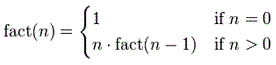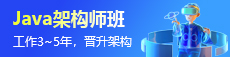# matlab递归调用的例子

## 1.基础

``````function y = ten_exp(n)
% 这是一个计算 y = 10^n 的递归程序。
% 该程序仅在 n 为非负整数时才有效。
% 如果 n 为负数，算法不会停止。
if n == 0
y = 1
else
n  %<< 此行不需要，但用于检查
y = 10 * ten_exp(n-1)
end``````

## 2.递归求解阶乘``````function y = fact(n)
% 我们有最大的数
y = n
%如果n == 0
y = 1则  我们下降到 0